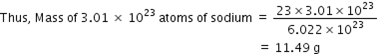Courses

# Test: Mole Concept

## 15 Questions MCQ Test Science Class 9 | Test: Mole Concept

Description
This mock test of Test: Mole Concept for Class 9 helps you for every Class 9 entrance exam. This contains 15 Multiple Choice Questions for Class 9 Test: Mole Concept (mcq) to study with solutions a complete question bank. The solved questions answers in this Test: Mole Concept quiz give you a good mix of easy questions and tough questions. Class 9 students definitely take this Test: Mole Concept exercise for a better result in the exam. You can find other Test: Mole Concept extra questions, long questions & short questions for Class 9 on EduRev as well by searching above.
QUESTION: 1

### The total number of atoms represented by the compound CuSO4.5H2O is -

Solution:

Copper atom(s) = 1
Sulfur atom(s) = 1
Oxygen atoms = 4 + 5 = 9
Hydrogen atoms = 10

Total atoms = 1 + 1 + 9 + 10 = 21

∴ There are total 21 atoms in CuSO₄·5H₂O (also called blue vitriol).

QUESTION: 2

### If 12 grams of carbon has n atoms, then the number of atoms in 12 grams of magnesium will be:

Solution:

C =  12 g = 1 mole
1 mole = 24 g = n atoms
1 g = (n  * 12) / 24
1 g  =  n /2

QUESTION: 3

### What is the value of Avogadro’s number?

Solution:

Avogadro’s number, number of units in one mole of any substance (defined as its molecular weightin grams), equal to 6.022140857 × 1023.

QUESTION: 4

1 mole of water molecules has:

Solution:

Water (H2O) is made from 2 atoms of hydrogen and 1 atom of oxygen. A mole of water molecules would be 2 moles of hydrogen atoms plus 1 mole of oxygen atoms.

QUESTION: 5

How many atoms does one mole of Phosphorus molecule contain?

Solution:

Phosphorus exists as a tetratomic molecule i.e.  Pmolecule. Thus, 1 mole of P4 = 4 × 6.022 × 1023 atoms

QUESTION: 6

Which of the following pair of elements represents a mole ratio of 1:1?

Solution:

14 g of nitrogen = gram atomic mass of nitrogen = 1 mole of nitrogen atoms

24 g of magnesium = gram atomic mass of magnesium = 1 mole of magnesium atoms

So, mole ratio = 1:1

QUESTION: 7

The number of moles of oxygen atoms in two moles of nitric acid is:

Solution:
QUESTION: 8

Identify the symbol used to represent Avogadro’s number.​

Solution:

Avogadro's number,NA,is defined as the number of atoms in 12 gram of carbon-12 atoms in their ground state at rest.

By definition it is related to the atomic mass constant  mu  by the relation.

Avogadro's number are defined in terms of the atomic mass constant (one twelfth of the mass of a 12C atom).

Avogadro's constant and number have by definition the same numerical value. In practice the two terms are used interchangeably.

QUESTION: 9

The mass of 0.5 moles of Water molecules is:(Atomic mass, H =1; O = 16)

Solution:

Given Data:
Mass of Water = ?
no. of moles = 0.5
Molar mass of H₂O = 2(1) + 16 = 18 g/mol

Formula to be Used:
No. of moles = Given Mass/Molar mass

Solution:
No. of moles = Mass of Water/Molar mass
0.5 = Mass of Water/18
Mass of Water = 0.5*18
Mass of Water = 9g

QUESTION: 10

The number of moles present in 52g of He(Atomic mass, He = 4) are:

Solution:

no of moles = mass in gram / molecular mass

no. of moles = 52/4 =13

QUESTION: 11

How many atoms are present in SO42- ion ?

Solution:

A sulphate ion (SO42-) contains one sulphur atom and four oxygen atoms. Therefore there are 5 atoms in a sulphate ion. In the ammonium ion (NH4+) there is one nitrogen atom and four hydrogen atoms. So, this ion also contains 5 atoms.

QUESTION: 12

Calculate the relative molecular mass of H2S.

Solution:

Atomic mass of hydrogen = 1 a.m.u.

Atomic mass of Sulphur = 32 a.m.u.

Thus, the molecular mass of hydrogen sulphide, which contains two atoms of hydrogen and one atom of sulphur is = 2 × 1 + 1 × 32 = 34 a.m.u.

QUESTION: 13

If atomic mass of sodium is 23 a.m.u., then the mass of 3.01 x 1023 sodium atoms is:

Solution:

Mass of 6.022 X 10 23 atoms (1 mole) of sodium = 23 gQUESTION: 14

The atomic mass of calcium is 40. Calculate the number of moles in 16 grams of calcium.

Solution:

Number of moles of Ca :-
n = Given mass in grams / molar mass
=   16 / 40
=   0.4 mole.

QUESTION: 15

One mole of molecules may not be equal to:​

Solution:

- The mole allows scientists to calculate the number of elementary entities (usually atoms or molecules) in a certain mass of a given substance.

- Avogadro’s number is an absolute number: there are 6.022x10^23 elementary entities in 1 mole. This can also be written as 6.022x10^23 mol-1.

- The mass of one mole of a substance is equal to that substance’s molecular weight. For example, the mean molecular weight of water is 18.015 atomic mass units (amu), so one mole of water weight 18.015 grams.